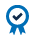##### Education Home

Futures contracts can be an effective and efficient risk management or trading tool. Their performance is basically two-dimensional, either you are up money or down depending on the entry price point and whether the market is up or down versus your position.

But with options on futures there are more dimension, or forces, acting on the price or premium of the option.

There are metrics to measure each of these different forces impacts on the premium of an options. These metrics are often referred to by their Greek letter and collectively known as the Greeks.

## Gamma

We discussed previously that delta is the change in the options price or premium due to the change in the underlying futures contract price.

We will now discuss how delta itself changes with a change in the underlying futures price. This is known as gamma. Think of gamma as the delta of the delta.

Look at it a different way; you are driving a car at 30 miles per hour (mph). If you increase your speed to 40 mph, you have accelerated by 10 miles per hour. If you think of speed as your delta, then the change in your speed is your gamma. In other words, gamma is your acceleration.

## Understanding Gamma Movements

Gamma is usually expressed as a change in the delta per one point change in the price of the underlying.

For example, if the futures price is 200, a 220 call has a delta of 30 and a gamma of 2.

If the futures price increases to 201, the delta is now 32. Conversely, if the futures price decreased to 199, the delta is 28.

Just like delta, gamma is dynamic. It is the highest when the underlying price is near the option’s strike price.

As the underlying moves away from the strike price, the gamma decreases. As the underlying moves towards the strike price, the gamma increases.

At the money options have the highest gamma, because their deltas are the most sensitive to underlying price changes.

## Calculating Gamma

Gamma is the difference in delta divided by the change in underlying price.

You have an underlying futures contract at 200 and the strike is 200. The options delta is 50 and the options gamma is 3. If the futures price moves to 201, the options delta is changes to 53. If the futures price moves down to 199, the options delta is 47.

Across the two-point underlying futures contract move, the delta changed by 6.

If you are going to trade options, Gamma is a measurement you will want to study.

#### ACCREDITED COURSEDid you know that CME Institute classes can fulfill CFA and GARP continuing education requirements? Every CME Institute course can be self-reported in your CFA online CE tracker and select classes can be used for GARP credits. See which of our classes qualify for GARP credits here.